# Unbalanced Forces

## Key Stage 3

### Meaning

Unbalanced Forces are Forces acting in opposite directions with different magnitudes.

When there are unbalanced forces on an object the object will accelerate.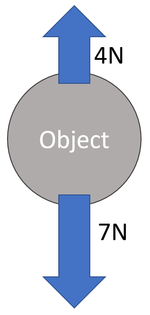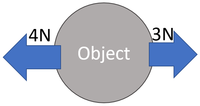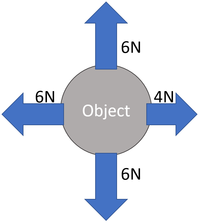The 4N up and the 7N down mean the object will accelerate downwards due to a resultant force of 3N. The 4N left and the 3N right mean the object will accelerate to the left due to a resultant force of 1N. The 6N up is balanced by the 6N down but the 6N left and the 4N right mean the object will accelerate to the left due to a resultant force of 2N.

## Key Stage 4

### Meaning

Unbalanced forces are force vectors which add together to make a (non-zero) resultant force.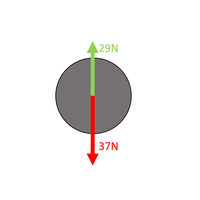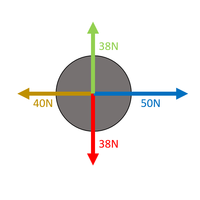Choose which directions are positive. Up is positive. Calculate the Resultant Force There is 29N up and 37N down. FR = (+29N) + (-37N) FR = -8N upwards The forces are unbalanced. Choose which directions are positive. Right is positive. Calculate the Resultant Force There is 114N right and 105N left. FR = (+114N) + (-105N) FR = 9N right The forces are unbalanced. Choose which directions are positive. Right is positive. Up is positive. Calculate the Resultant Force There is 38N up and 38N down. FR = (+38N) + (-38N) FR = 0N upwards The vertical forces are balanced. Calculate the Resultant Force There is 50N right and 40N left. FR = (+50N) + (-40N) FR = 10N right The horizontal forces are unbalanced.